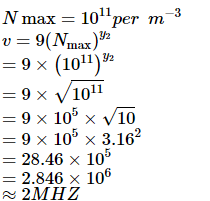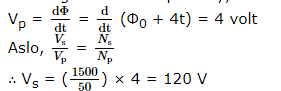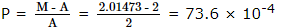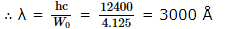Courses

# VITEEE Physics Test - 9

## 40 Questions MCQ Test VITEEE: Subject Wise and Full Length MOCK Tests | VITEEE Physics Test - 9

Description
This mock test of VITEEE Physics Test - 9 for JEE helps you for every JEE entrance exam. This contains 40 Multiple Choice Questions for JEE VITEEE Physics Test - 9 (mcq) to study with solutions a complete question bank. The solved questions answers in this VITEEE Physics Test - 9 quiz give you a good mix of easy questions and tough questions. JEE students definitely take this VITEEE Physics Test - 9 exercise for a better result in the exam. You can find other VITEEE Physics Test - 9 extra questions, long questions & short questions for JEE on EduRev as well by searching above.
QUESTION: 1

Solution:
QUESTION: 2

Solution:
QUESTION: 3

### The wavelength of X - rays is of the order of

Solution:
QUESTION: 4

The negative Zn pole of Daniell cell, sending a constant current through a circuit, decreases in mass by 0.13g in 30 min. If the electrochemical equivalent of Zn and Cu are 32.5 and 31.5 respectively, the increase in the mass of the positive Cu pole in this time is

Solution:
QUESTION: 5

Two ions having masses in the ratio 1 : 1 and charges 1 : 2 are projected into uniform magnetic field perpendicular to the field with speeds in the ratio 2 : 3. The ratio of the radii of circular paths along which the two particles move is

Solution:
QUESTION: 6

Two identical cells each of emf E and internal resistance r are connected in parallel with an external resistance R. To get maximum power developed across R, the value of R is

Solution:

According to max. power transfer theorem, power transferred to the load will be maximum when load resistance is equal to internal resistance
i.e., R = r/2

QUESTION: 7

In short wave communication waves of which of the following frequencies will be reflected back by the ionospheric layer having electron density 1011 per m-3?

Solution:QUESTION: 8

In a potentiometer, the null point is received at 7th wire. If now we have to change the null point at 9th wire, what should we do?

Solution:
QUESTION: 9

If two identical heaters of 220 V, 1000 W are placed parallel to each other across 220 V lines, then the combined power of the heaters is

Solution:
QUESTION: 10
A galvanometer of 100 Ω resistance gives full scale deflection when 10 mA of current is passed. To convert it into 10 A range ammeter, the resistance of the shunt required will be
Solution:
QUESTION: 11
The work function of a substance is 4 eV. The longest wavelength of light that can cause photoelectron emission from this substance is approximetely
Solution:
QUESTION: 12

A wheel having metal spokes of 1m long between its axle and rim is rotating in a magnetic field of flux density 5x10-5 T normal to the plane of the wheel. An e.m.f of 22/7 mV is produced between the rim and the axle of the wheel. The rate of rotation of the wheel in radians per second is

Solution:
QUESTION: 13

The primary and secondary coils of a transformer have 50 and 1500 turns respectively. If the magnetic flux φ linked with the primary coil is given by φ = φ 0 + 4t, where φ is in weber, t is time in second and φ 0 is a constant, the output voltage across the secondary coil is

Solution:

No. of turns across primary Np = 50
Number of turns across secondary Ns = 1500
Magnetic flux linked with primary, Φ = Φ0 + 4t
∴ Voltage across the primary,QUESTION: 14
Which of the following figures correctly depicts the Lenz's law? The arrows show the movement of the labelled pole of a bar magnet into a closed circular loop and the arrows on the circle show the direction of the induced current.
Solution:
QUESTION: 15

Eight dipoles of charges of magnitude e are placed inside a cube. The total electric flux coming out of the cube will be

Solution:
QUESTION: 16

Heat radiations propagate with the speed of

Solution:
QUESTION: 17

A hollow metal sphere of radious 10 cm is charged such that the potential on its surface is 80V. The potential at the centre of the sphere is

Solution:
QUESTION: 18

In bringing an electron towards another electron, the electrostatic potential energy of the system

Solution:
QUESTION: 19

The capacitance of a parallel plate capacitor is 10 μF when distance between its plates is 8 cm. If distance between the plates is reduced to 4 cm, its capacitance will be

Solution:
QUESTION: 20

A solid conducting sphere having a charge Q is surrounded by an uncharged concentric conducting hollow spherical shell. The potential difference between the surfaces of solid sphere and hollow shell is V. If the shell is now given a charge of -3Q, then the new potential difference between the two surfaces will be

Solution:
QUESTION: 21

In radioactive decay process, the negatively charged emitted β -particles are

Solution:
QUESTION: 22

A straight wire of length 0.5 meter and carrying a current of 1.2 ampere is placed in a uniform magnetic field of induction 2 telsa. The magnetic field is perpendicular to the length of the wire. The force on the wire is

Solution:
QUESTION: 23

A thin spherical conducting shell of radius R has charge q. Another charge Q is placed at the centre of the shell. The electrostatic potential at a point P at a distance R/2 from the centre of the shell is

Solution:
QUESTION: 24

The masses of neutron, proton and deuteron in a.m.u are 1.00893 1.00813 and 2.01473 respectively. The packing fraction of the deuteron in a.m.u is

Solution:

Packing fraction = (M - A)/A , where M is the atomic mass and A is the mass number.QUESTION: 25

The energy released per fission of a U235 nucleus is around

Solution:
QUESTION: 26

A photon of energy 3.4 eV is incident on a metal having work function 2 eV. The maximum K.E. of photo-electrons is equal to

Solution:
QUESTION: 27

The photoelectric work function for a metal surface is 4.125 eV. The cut off wavelength for this surface is

Solution:

Work function W0 = hc/λQUESTION: 28

The refractive index of glass is 1.520 for red light and 1.525 for blue light. Let D1 and D2 be angels of minimum deviation for red and blue light respectively in a prism of this glass, then,

Solution:
QUESTION: 29

In visible region the dispersive powers and the mean angular deviations for crown and flint glass prisms are ω , ω ' and d, d' respectively. The condition for getting dispersion with zero deviation, when the two prisms are combined is

Solution:
QUESTION: 30

Fermi level in an intrinsic semiconductor lies

Solution:
QUESTION: 31

In an n-p-n transistor

Solution:
QUESTION: 32

In an n-p-n transistor, p-acts as a/an

Solution:
QUESTION: 33

N-type semiconductors will be obtained, when germanium is doped with

Solution:
QUESTION: 34

When the P end of P-N junction is connected to the negative terminal of the battery and the N end to the positive terminal of the battery, then the P-N junction behaves like

Solution:
QUESTION: 35

The valency of an impurity added to germanium crystal in order to convert it into a P-type semi-conductor is

Solution:
QUESTION: 36

In N-type semiconductors, majority charge carriers are

Solution:
QUESTION: 37

Laser light is considered to be coherent as it consists of

Solution:
QUESTION: 38

In young's double slit experiment, the intensity of central maximum is I. It one slit is closed, the intensity at the same place will become

Solution:
QUESTION: 39

A ray of light falls on a transparent glass slab of refractive index 1.62. If the reflected ray and the refracted ray are mutually perpendicular, the angle of incidence is

Solution:
QUESTION: 40

In Young's double slit interference experiment the wavelength of light used is 6000 Å . If the path difference between waves reaching a point P on the screen is 1.5 microns, then at that point P

Solution: